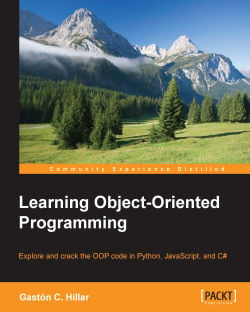### Learning Object-Oriented Programming4.5 (2 reviews total)
By Gaston C. Hillar

• Constantly updated with 100+ new titles each month
• Breadth and depth in over 1,000+ technologies

Learning Object-Oriented Programming is an easy-to-follow guide full of hands-on examples of solutions to common problems with object-oriented code in Python, JavaScript, and C#. It starts by helping you to recognize objects from real-life scenarios and demonstrates that working with them makes it simpler to write code that is easy to understand and reuse. You will learn to protect and hide data with the data encapsulation features of Python, JavaScript, and C#.

You will explore how to maximize code reuse by writing code capable of working with objects of different types, and discover the advantage of duck typing in both Python and JavaScript, while you work with interfaces and generics in C#. With a fair understanding of interfaces, multiple inheritance, and composition, you will move on to refactor existing code and to organize your source for easy maintenance and extension.

Learning Object-Oriented Programming will help you to make better, stronger, and reusable code.

Publication date:
July 2015
Publisher
Packt
Pages
280
ISBN
9781785289637

## Chapter 1. Objects Everywhere

Objects are everywhere, and therefore, it is very important to recognize elements, known as objects, from real-world situations. It is also important to understand how they can easily be translated into object-oriented code. In this chapter, you will learn the principles of object-oriented paradigms and some of the differences in the approaches towards object-oriented code in each of the three programming languages: Python, JavaScript, and C#. In this chapter, we will:

• Understand how real-world objects can become a part of fundamental elements in the code

• Recognize objects from nouns

• Generate blueprints for objects and understand classes

• Recognize attributes to generate fields

• Recognize actions from verbs to generate methods

• Work with UML diagrams and translate them into object-oriented code

• Organize blueprints to generate different classes

• Identify the object-oriented approaches in Python, JavaScript, and C#

## Recognizing objects from nouns

Let's imagine, we have to develop a new simple application, and we receive a description with the requirements. The application must allow users to calculate the areas and perimeters of squares, rectangles, circles, and ellipses.

It is indeed a very simple application, and you can start writing code in Python, JavaScript, and C#. You can create four functions that calculate the areas of the shapes mentioned earlier. Moreover, you can create four additional functions that calculate the perimeters for them. For example, the following seven functions would do the job:

• `calculateSquareArea`: This receives the parameters of the square and returns the value of the calculated area for the shape

• `calculateRectangleArea`: This receives the parameters of the rectangle and returns the value of the calculated area for the shape

• `calculateCircleArea`: This receives the parameters of the circle and returns the value of the calculated area for the shape

• `calculateEllipseArea`: This receives the parameters of the ellipse and returns the value of the calculated area for the shape

• `calculateSquarePerimeter`: This receives the parameters of the square and returns the value of the calculated perimeter for the shape

• `calculateRectanglePerimeter`: This receives the parameters of the rectangle and returns the value of the calculated perimeter for the shape

• `calculateCirclePerimeter`: This receives the parameters of the circle and returns the value of the calculated perimeter for the shape

However, let's forget a bit about programming languages and functions. Let's recognize the real-world objects from the application's requirements. It is necessary to calculate the areas and perimeters of four elements, that is, four nouns in the requirements that represent real-life objects:

• Square

• Rectangle

• Circle

• Ellipse

We can design our application by following an object-oriented paradigm. Instead of creating a set of functions that perform the required tasks, we can create software objects that represent the state and behavior of a square, rectangle, circle, and an ellipse. This way, the different objects mimic the real-world shapes. We can work with the objects to specify the different attributes required to calculate their areas and their perimeters.

Now, let's move to the real world and think about the four shapes. Imagine that you have to draw the four shapes on paper and calculate both their areas and perimeters. What information do you require for each of the shapes? Think about this, and then, take a look at the following table that summarizes the data required for each shape:

Shape

Required data

Square

Length of side

Rectangle

Width and height

Circle

Ellipse

Semi-major axis (usually labeled as a) and semi-minor axis (usually labeled as b)

### Tip

The data required by each of the shapes is going to be encapsulated in each object. For example, the object that represents a rectangle encapsulates both the rectangle's width and height. Data encapsulation is one of the major pillars of object-oriented programming.

The following diagram shows the four shapes drawn and their elements: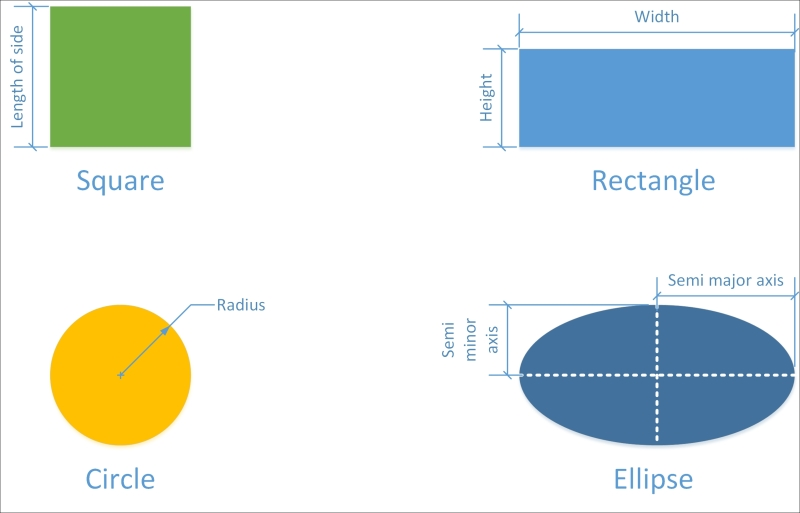## Generating blueprints for objects

Imagine that you want to draw and calculate the areas of four different rectangles. You will end up with four rectangles drawn, with their different widths, heights, and calculated areas. It would be great to have a blueprint to simplify the process of drawing each rectangle with their different widths and heights.

In object-oriented programming, a class is a blueprint or a template definition from which the objects are created. Classes are models that define the state and behavior of an object. After defining a class that defines the state and behavior of a rectangle, we can use it to generate objects that represent the state and behavior of each real-world rectangle.

### Tip

Objects are also known as instances. For example, we can say each rectangle object is an instance of the rectangle class.

The following image shows four rectangle instances drawn, with their widths and heights specified: Rectangle #1, Rectangle #2, Rectangle #3, and Rectangle #4. We can use a `rectangle` class as a blueprint to generate the four different `rectangle` instances. It is very important to understand the difference between a class and the objects or instances generated through its usage. Object-oriented programming allows us to discover the blueprint we used to generate a specific object. Thus, we are able to infer that each object is an instance of the `rectangle` class.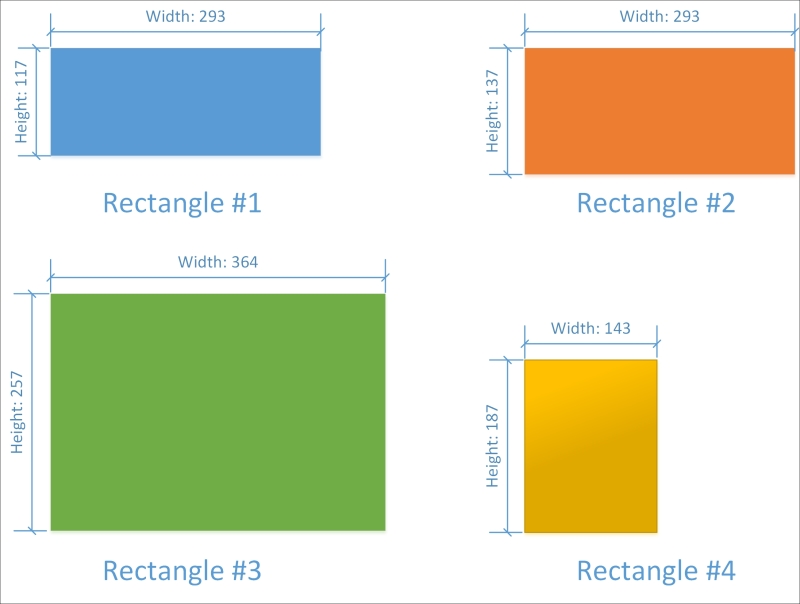We recognized four completely different real-world objects from the application's requirements. We need classes to create the objects, and therefore, we require the following four classes:

• Square

• Rectangle

• Circle

• Ellipse

## Recognizing attributes/fields

We already know the information required for each of the shapes. Now, it is time to design the classes to include the necessary attributes that provide the required data to each instance. In other words, we have to make sure that each class has the necessary variables that encapsulate all the data required by the objects to perform all the tasks.

Let's start with the Square class. It is necessary to know the length of side for each instance of this class, that is, for each `square` object. Thus, we need an encapsulated variable that allows each instance of this class to specify the value of the length of side.

### Tip

The variables defined in a class to encapsulate data for each instance of the class are known as attributes or fields. Each instance has its own independent value for the attributes or fields defined in the class.

The `Square` class defines a floating point attribute named `LengthOfSide` whose initial value is equal to `0` for any new instance of the class. After you create an instance of the `Square` class, it is possible to change the value of the `LengthOfSide` attribute.

For example, imagine that you create two instances of the `Square` class. One of the instances is named square1, and the other is square2. The instance names allow you to access the encapsulated data for each object, and therefore, you can use them to change the values of the exposed attributes.

Imagine that our object-oriented programming language uses a dot (`.`) to allow us to access the attributes of the instances. So, `square1.LengthOfSide` provides access to the length of side for the `Square` instance named `square1`, and `square2.LengthOfSide` does the same for the `Square` instance named `square2`.

You can assign the value `10` to `square1.LengthOfSide` and `20` to `square2.LengthOfSide`. This way, each `Square` instance is going to have a different value for the `LengthOfSide` attribute.

Now, let's move to the Rectangle class. We can define two floating-point attributes for this class: `Width` and `Height`. Their initial values are also going to be `0`. Then, you can create two instances of the `Rectangle` class: `rectangle1` and rectangle2.

You can assign the value `10` to `rectangle1.Width` and `20` to `rectangle1.Height`. This way, `rectangle1` represents a 10 x 20 rectangle. You can assign the value `30` to` rectangle2.Width` and `50` to `rectangle2.Height` to make the second `Rectangle` instance, which represents a 30 x 50 rectangle.

The following table summarizes the floating-point attributes defined for each class:

Class name

Attributes list

Square

LengthOfSide

Rectangle

Width

Height

Circle

Ellipse

SemiMajorAxis

The following image shows a UML (Unified Modeling Language) diagram with the four classes and their attributes: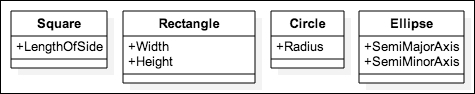## Recognizing actions from verbs – methods

So far, we have designed four classes and identified the necessary attributes for each of them. Now, it is time to add the necessary pieces of code that work with the previously defined attributes to perform all the tasks. In other words, we have to make sure that each class has the necessary encapsulated functions that process the attribute values specified in the objects to perform all the tasks.

Let's start with the Square class. The application's requirements specified that we have to calculate the areas and perimeters of squares. Thus, we need pieces of code that allow each instance of this class to use the LengthOfSide value to calculate the area and the perimeter.

### Tip

The functions or subroutines defined in a class to encapsulate the behavior for each instance of the class are known as methods. Each instance can access the set of methods exposed by the class. The code specified in a method is able to work with the attributes specified in the class. When we execute a method, it will use the attributes of the specific instance. A good practice is to define the methods in a logical place, that is, in the place where the required data is kept.

The `Square` class defines the following two parameterless methods. Notice that we declare the code for both methods in the definition of the `Square` class:

• `CalculateArea`: This returns a floating-point value with the calculated area for the square. The method returns the square of the `LengthOfSide` attribute value (LengthOfSide2 or LengthOfSide ^ 2).

• `CalculatePerimeter`: This returns a floating-point value with the calculated perimeter for the square. The method returns the `LengthOfSide` attribute value multiplied by `4` (4 * LengthOfSide).

Imagine that, our object-oriented programming language uses a dot (`.`) to allow us to execute methods of the instances. Remember that we had two instances of the `Square` class: `square1` with `LengthOfSide` equal to `10` and `square2` with `LengthOfSide` equal to `20`. If we call `square1.CalculateArea`, it would return the result of 102, which is `100`. On the other hand, if we call `square2.CalculateArea`, it would return the result of 202, which is `400`. Each instance has a diverse value for the `LengthOfSide` attribute, and therefore, the results of executing the `CalculateArea` method are different.

If we call `square1.CalculatePerimeter`, it would return the result of 4 * 10, which is `40`. On the other hand, if we call `square2.CalculatePerimeter`, it would return the result of 4 * 20, which is `80`.

Now, let's move to the Rectangle class. We need exactly two methods with the same names specified for the `Square` class. However, they have to calculate the results in a different way.

• `CalculateArea`: This returns a floating-point value with the calculated area for the rectangle. The method returns the result of the multiplication of the `Width` attribute value by the `Height` attribute value (Width * Height).

• `CalculatePerimeter`: This returns a floating-point value with the calculated perimeter for the rectangle. The method returns the sum of two times the `Width` attribute value and two times the `Height` attribute value (2 * Width + 2 * Height).

Remember that, we had two instances of the `Rectangle` class: `rectangle1` representing a 10 x 20 rectangle and `rectangle2` representing a 30 x 50 rectangle. If we call `rectangle1.CalculateArea`, it would return the result of 10 * 20, which is `200`. On the other hand, if we call `rectangle2.CalculateArea`, it would return the result of 30 * 50, which is `1500`. Each instance has a diverse value for both the `Width` and `Height` attributes, and therefore, the results of executing the `CalculateArea` method are different.

If we call `rectangle1.CalculatePerimeter`, it would return the result of 2 * 10 + 2 * 20, which is `60`. On the other hand, if we call `rectangle2. CalculatePerimeter`, it would return the result of 2 * 30 + 2 * 50, which is `160`.

The Circle class also needs two methods with the same names. The two methods are explained as follows:

• `CalculateArea`: This returns a floating-point value with the calculated area for the circle. The method returns the result of the multiplication of π by the square of the `Radius` attribute value (π * Radius2 or π * (Radius ^ 2)).

• `CalculatePerimeter`: This returns a floating-point value with the calculated perimeter for the circle. The method returns the result of the multiplication of π by two times the `Radius` attribute value.

Finally, the Ellipse class defines two methods with the same names but with different code and a specific problem with the perimeter. The following are the two methods:

• `CalculateArea`: This returns a floating-point value with the calculated area for the ellipse. The method returns the result of the multiplication of π by the square of the `Radius` attribute value (π * SemiMajorAxis * SemiMinorAxis).

• `CalculatePerimeter`: This returns a floating-point value with the calculated approximation of the perimeter for the ellipse. Perimeters are very difficult to calculate for ellipses, and therefore, there are many formulas that provide approximations. An exact formula needs an infinite series of calculations. Thus, let's consider that the method returns the result of a formula that isn't very accurate and that we will have to improve on it later. The method returns the result of 2 * π * SquareRoot ((SemiMajorAxis2 + SemiMinorAxis2) / 2).

The following figure shows an updated version of the UML diagram with the four classes, their attributes, and their methods: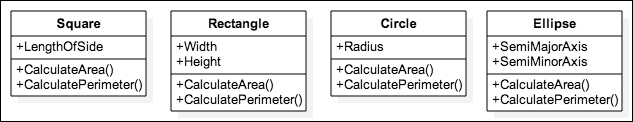## Organizing the blueprints – classes

So far, our object-oriented solution includes four classes with their attributes and methods. However, if we take another look at these four classes, we would notice that all of them have the same two methods: `CalculateArea` and `CalculatePerimeter`. The code for the methods in each class is different, because each shape uses a different formula to calculate either the area or the perimeter. However, the declarations or the contracts for the methods are the same. Both methods have the same name, are always parameterless, and both return a floating-point value.

When we talked about the four classes, we said we were talking about four different geometrical shapes or simply, shapes. Thus, we can generalize the required behavior for the four shapes. The four shapes must declare the `CalculateArea` and `CalculatePerimeter` methods with the previously explained declarations. We can create a contract to make sure that the four classes provide the required behavior.

The contract will be a class named `Shape`, and it will generalize the requirements for the geometrical shapes in our application. The `Shape` class declares two parameterless methods that return a floating-point value: `CalculateArea` and `CalculatePerimeter`. Then, we can declare the four classes as subclasses of the Shape class that inherit these definitions, but provide the specific code for each of these methods.

### Tip

We can define the `Shape` class as an abstract class, because we don't want to be able to create instances of this class. We want to be able to create instances of `Square`, `Rectangle`, `Circle`, or `Ellipse`. In this case, the `Shape` abstract class declares two abstract methods. We call `CalculateArea` and `CalculatePerimeter` abstract methods because the abstract class declares them without an implementation, that is, without code. The subclasses of `Shape` implement the methods because they provide code while maintaining the same method declarations specified in the `Shape` superclass. Abstraction and hierarchy are the two major pillars of object-oriented programming.

Object-oriented programming allows us to discover whether an object is an instance of a specific superclass. After we changed the organization of the four classes and they became subclasses of the `Shape` class, any instance of `Square`, `Rectangle`, `Circle`, or `Ellipse` is also an instance of the `Shape` class. In fact, it isn't difficult to explain the abstraction because we are telling the truth about the object-oriented model that represents the real world. It makes sense to say that a rectangle is indeed a shape, and therefore, an instance of a `Rectangle` class is a `Shape` class. An instance of a `Rectangle` class is both a `Shape` class (the superclass of the `Rectangle` class) and a `Rectangle` class (the class that we used to create the object).

When we were implementing the `Ellipse` class, we discovered a specific problem for this shape; there are many formulas that provide approximations of the perimeter value. Thus, it makes sense to add additional methods that calculate the perimeter using other formulas.

We can define the following two additional parameterless methods, that is, two methods without any parameter. These methods return a floating-point value to the `Ellipse` class to solve the specific problem of the ellipse shape. The following are the two methods:

• `CalculatePerimeterWithRamanujanII`: This uses the second version of a formula developed by Srinivasa Aiyangar Ramanujan

• `CalculatePerimeterWithCantrell`: This uses a formula proposed by David W. Cantrell

This way, the `Ellipse` class implements the methods specified in the `Shape` superclass. The `Ellipse` class also adds two specific methods that aren't included in any of the other subclasses of `Shape`.

The following diagram shows an updated version of the UML diagram with the abstract class, its four subclasses, their attributes, and their methods: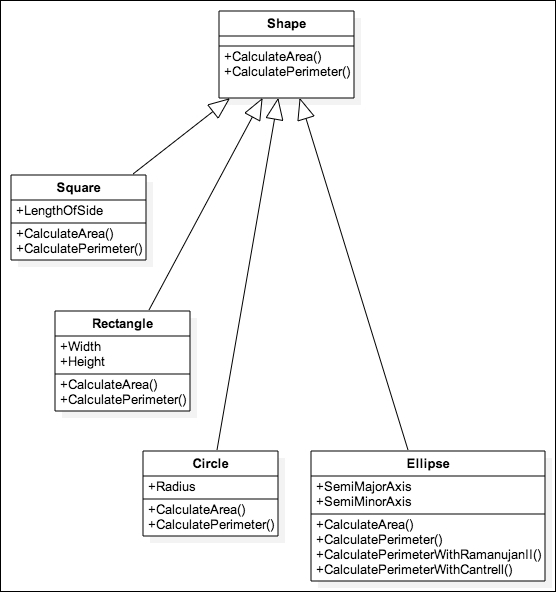## Object-oriented approaches in Python, JavaScript, and C#

Python, JavaScript, and C# support object-oriented programming, also known as OOP. However, each programming language takes a different approach. Both Python and C# support classes and inheritance. Therefore, you can use the different syntax provided by each of these programming languages to declare the `Shape` class and its four subclasses. Then, you can create instances of each of the subclasses and call the different methods.

On the other hand, JavaScript uses an object-oriented model that doesn't use classes. This object-oriented model is known as prototype-based programming. However, don't worry. Everything you have learned so far in your simple object-oriented design journey can be coded in JavaScript. Instead of using inheritance to achieve behavior reuse, we can expand upon existing objects. Thus, we can say that objects serve as prototypes in JavaScript. Instead of focusing on classes, we work with instances and decorate them to emulate inheritance in class-based languages.

### Tip

The object-oriented model known as prototype-based programing is also known by other names such as classless programming, instance-based programming, or prototype-oriented programming.

There are other important differences between Python, JavaScript, and C#. They have a great impact on the way you can code object-oriented designs. However, you will learn different ways throughout this book to make it possible to code the same object-oriented design in the three programming languages.

## Summary

In this chapter, you learned how to recognize real-world elements and translate them into the different components of the object-oriented paradigm: classes, attributes, methods, and instances. You understood the differences between classes (blueprints or templates) and the objects (instances). We designed a few classes with attributes and methods that represented blueprints for real-life objects. Then, we improved the initial design by taking advantage of the power of abstraction, and we specialized in the `Ellipse` class.

Now that you have learned some of the basics of the object-oriented paradigm, you are ready to start creating classes and instances in Python, JavaScript, and C# in the next chapter.